# Solve the “Planning Problem” with Integer Programming

Solving the “Planning Problem” with Integer Programming Photo by Estée Janssens on Unsplash This is a technical introduction to solving the Planning problem of a Workforce Scheduler. For more details about what the Scheduler is and why Jobandtalent have built such a product, check out the previous article: Save Money and Increase Workers Happiness by Optimising your […]

### Solving the “Planning Problem” with Integer Programming

This is a technical introduction to solving the Planning problem of a Workforce Scheduler. For more details about what the Scheduler is and why Jobandtalent have built such a product, check out the previous article:

Save Money and Increase Workers Happiness by Optimising your Workforce Schedule (1/2)

### The Whole Picture

What do we know?
We have a given demand of workforce over a certain period of time, a set of constraints that we need to respect in order to have a valid solution, and a set of preferences and costs that we want to optimize.

(a) Workforce demand: you can think of the demand as a list that states how many workers are needed in each hour.

(b) Constraints: the conditions that need to be satisfied to have a valid solution (shift size, shift type: continuous hours or with breaks, etc.).

(c) Preference and costs: the “preferences” of the workers or the clients
(e.g., ideal number of hours per shift), and the cost of oversupply and undersupply, number of active workers, etc. We will formulate both of these concepts into our Cost Function.

### Quick Introduction to LP, IP, and Solvers

As we mentioned in the previous article, we are dealing with a Discrete Optimization problem because we need to search for the optimal solution among a finite set of candidate solutions (which could be quite large).

With a bit of mathematics, we can describe the problem using Linear Integer Programming and run the search with one of the Open Source Solvers.

What is an “Integer Programming” Problem?
It’s a specific kind of Linear Programming (LP) or Linear Optimization. In short, it is a method that tries to find the best solutions to a mathematical model whose constraints are represented by linear relationships. In our scenario, all of the variables are restricted to be integers, and this is a special case which is called (Linear) Integer Programming (LIP or IP).

Once we design the IP problem we can run a Solver to search for the solution.

What is a “Solver”?
It’s a very efficient piece of software that is able to interpret mathematical optimization models, navigating their search space applying a variety of mathematical tricks to find the solution in the minimum possible time.

The best available Solvers number only a few, you can count them on two hands. The most critical part is how to design the model, and that is where your creativity and ability play a key role. You can define the variables you want, their interactions, how they can be mapped with the constraints, how they are combined to build the cost function, and so on.

### Mathematical Formulation

Mathematical formulation is just a way to define the problems with variables and operations. You need to define what the basic elements of the model you are building are, such as the hours, the demand, the shifts, and you can think of them as the players of the mathematical model.

Once we know what these players are, we need to translate the relationship between them into mathematical formulas using classic operations, mapping them with the problem constraints, then creating the model.

Let’s think about a simple Knapsack Problem.

We want to pack our small 20L backpack with different books (which have different volumes) that we can sell at different prices. The idea is to select them to fit inside the backpack capacity while maximizing their values (better to sells 5 30€ books, than 10 5€ ones).

The players of our story are simple:

• We have n books, that we can define as n variables {x1, x2, …, xn} which can be equal to 1 when the book is selected, or 0 when it is left at home.
• Each book has a volume (for simplicity we assume the shape is not relevant) and thus we have n other variables: {v1, v2, …, vn}
• And finally, we have the n prices: {p1, p2, …, pn}

We want to maximize the total possible profit (1) while respecting the maximum backpack capacity (2). And this would be our model:

If we select only books 1, 3, and 7, only the variables x1, x3, x7 will be non zero, and as a consequence, (1) will sum up only their prices (p1+p3+p7) and only their volumes will be considered in the inequality (2): v1+v3+v7 ≤ 10L and thus, just by playing with the x variables, we can mathematically compute and verify the cost function while checking that the constraint is respected.

That’s it.

We converted our real problem into a mathematical formulation that can be interpreted by a Solver.

### Model Design & Formulation

We are finally ready to talk about Jobandtalent’s case study: our Planning Algorithm.

Let’s consider a simple demand of 24 hours like the one below, from which we need to create the working shifts to associate with different workers.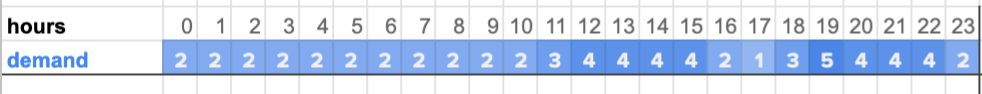For each slot between 0:00 until 23:00 the demand contains the number of workers that are needed, e.g., at 10:00 we need 2 workers, while in the following slots we need 3 (11:00) and 4 (12:00).

If you have to manually create these shifts, one very intuitive and visual approach is to extend this list with a matrix in which each row represents a shift (or worker) and each column contains the working status of that hour, when equal to 1 the shift is active, otherwise is not active. Then, we can play with the 1s and 0s, limiting the possible length of the shifts we generate, and ensuring that the 1s are all contiguous.

The image below should help you visualize it with a possible solution.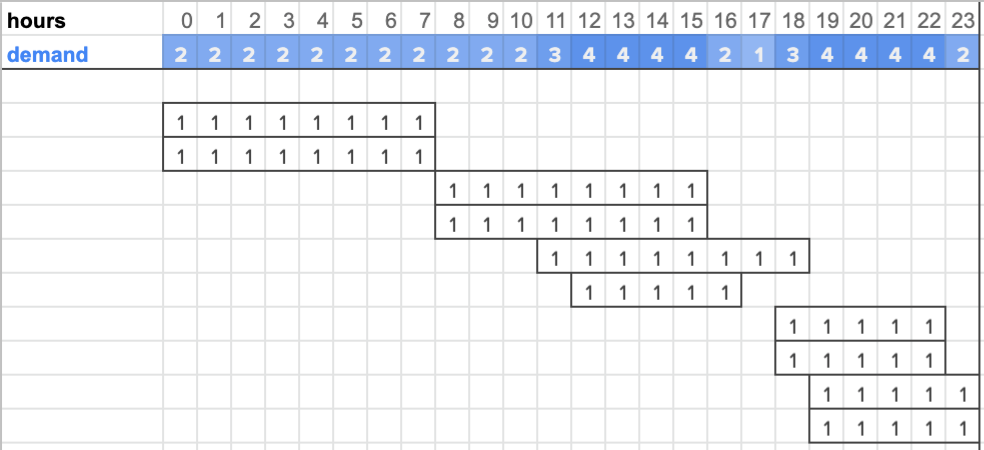This is a possible solution, following a simple matrix-based approach. With 10 shifts we can perfectly cover the 24h demand, and since all the shifts respect the constraints this solution is considered valid.

What we just did was think about a possible model or logic that could be used to find the solution. Now let’s see if we can formulate it mathematically.

First, let’s define the variables and constraints for our problem. We should start by defining the basic ones: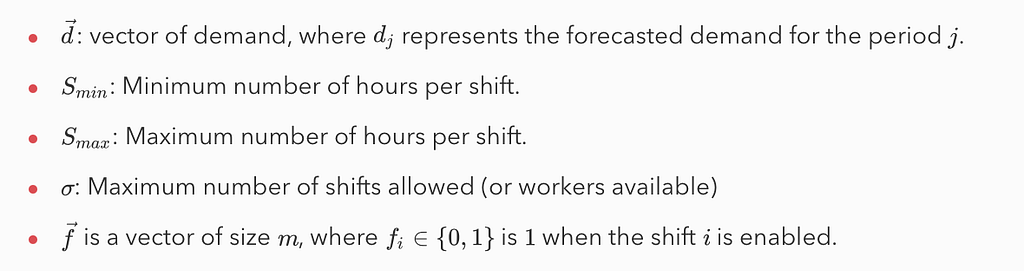These variables define the input demand, the min/max shift lengths, and the maximum number of available workers. Finally, the variable f keeps track of the assigned shifts, and this will be part of the cost function.

And the matrix: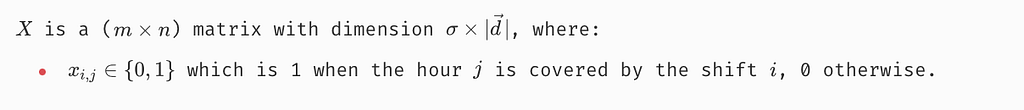The matrix contains in each cell a boolean variable that states if the shift (row) is supposed to be active in that specific hour (column). The matrix can be quite large, meaning that a lot of variables will be generated. However, since they are all boolean, the problem remains fairly simple to tackle.

After defining the first variables, we can already formulate some constraints.

In (1) we are stating that the solution we are looking for must contain shifts that cover exactly the given demand (1a). This is a very strict constraint because when a perfect solution doesn’t exist, it means that our Solver won’t return anything. If we want to be more flexible we could replace the equality operator with a “≥” or “≤” to allow oversupply (1b) or undersupply (1c).

In (4) and (5) we are stating that each shift must have a length that falls in the range of Smin and Smax (by summing up all the 1s each row contains). The variable f is used to force the inactive shifts to have 0s in all the rows of the matrix. The Solver, when looking for the solution, tries different permutations of fi always trying to optimize the cost function. For example, when it is checking f1=0 it is forcing all the integer column variables of the first shift to be 0 as well, in other words, it is disabling the first shift.

Contiguous Shifts Constraint
We have our matrix which tracks the activity hours of each possible shift. That’s great, but there is a big issue we have to solve now. How can we (mathematically) state that all the 1s of a row should be always contiguous?

Basically, the problem we have defined so far is not discarding the “uncontiguous shifts”, and we need to think about a constraint that will invalidate them making the Solver search for other solutions.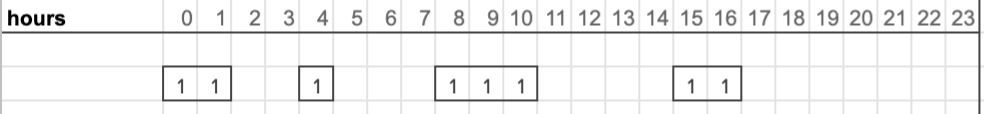That is an example of an “uncontiguous shift”. We should think about a constraint to avoid this solution.

One possible solution is to identify “when the shift starts”, which happens when we have a 0 followed by a 1 (keep in mind the particular case of the first hour). To keep track of this we need almost a variable for each cell, basically, we define another matrix of variables.

For the previous example, that specific row of the matrix C will look like this: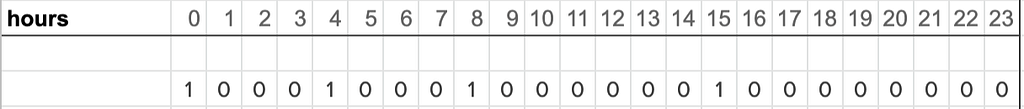The 1s mark the hour in which the shift starts. In this example, we can see how the matrix C is correctly counting four different starts. The next step is to add constraints that would mark this solution as invalid.

What we can do is count the number of times the “shift starts” in each row, basically when, taking two cells, the latter is bigger than the former, as shown in (7). Finally, in (8) we limit the sum to be lower or equal at 1, which means the shift is empty (no starting hours) or that it starts just once.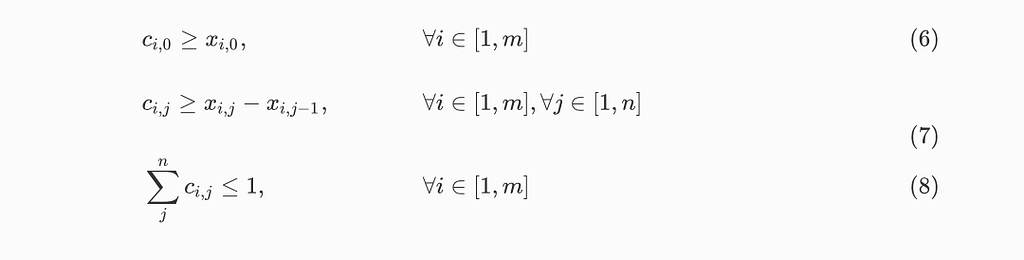Constraints of the matrix C, fundamental to force the shifts to be contiguous.

#### Objective function

Finally, our objective function has to minimize the cost of adding extra shifts. For simplicity, we can just minimize the number of shifts, so the sum of the fi.

If we have to find a solution that matches exactly the demand, basically using (1), this objective function is enough. Instead, if we used (1a) or (1b), we need to extend the objective function with additional costs: the number of oversupplied and/or undersupplied hours. In the final objective function (11) we can easily balance the importance of minimizing the shifts (9) and the over/undersupply (10).

Note that to calculate the over and undersupply in (10), we cannot use the absolute and max function since they are not linear and thus not compatible with Linear Programming. Instead, we need to use a little trick.

We have to create two non-negative variables, (12a) and (12b), and equalize their difference with the difference of hours allocated and demand for each column. For example, assume column 1 had a demand of 8 workers but our solution allocated 10, with this formula, because we are minimizing oversupply and undersupply, the Solver will automatically assign 0 to undersupply and 2 to oversupply, validating the equality.

And this is it.

We have built our objective function, the last piece of our model. Now we can run the Solver to search for the optimal solution to our problem.

#### Conclusions

In this article, we have discussed a simple Integer Programming model that is able to solve a wide set of generic Planning problems.

The model can become substantially more complex if extended with more complex preferences or requirements, such as:

• Oversupply/Undersupply weights. Having a worker that does more hours than the required has a certain cost. Not being able to cover the initial demand because we undersupply, often has a different cost.
• Dynamic weighting. A solution with 1 extra hour over 5 different time slots might be better than a solution with 5 extra hours in one single slot. One way to account for this difference is to have dynamic weightings that give a higher penalization to the “higher error” (i.e., check out the stepwise function since non-linear functions are always forbidden).
• Accepting shifts with breaks. What about if we can accept solutions with shifts that contain 1 or 2 hours of breaks? Well, we should find a way to add it to the C matrix, and if that’s not possible we have to think of a completely different model.

Adding more preferences means adding more variables and constraints to the model while ensuring they can work together. Moreover, note that all these extensions will make the search problem substantially more complex for the Solver.

### We are hiring!

body[data-twttr-rendered=”true”] {background-color: transparent;}.twitter-tweet {margin: auto !important;}

function notifyResize(height) {height = height ? height : document.documentElement.offsetHeight; var resized = false; if (window.donkey && donkey.resize) {donkey.resize(height);resized = true;}if (parent && parent._resizeIframe) {var obj = {iframe: window.frameElement, height: height}; parent._resizeIframe(obj); resized = true;}if (window.location && window.location.hash === “#amp=1” && window.parent && window.parent.postMessage) {window.parent.postMessage({sentinel: “amp”, type: “embed-size”, height: height}, “*”);}if (window.webkit && window.webkit.messageHandlers && window.webkit.messageHandlers.resize) {window.webkit.messageHandlers.resize.postMessage(height); resized = true;}return resized;}twttr.events.bind(‘rendered’, function (event) {notifyResize();}); twttr.events.bind(‘resize’, function (event) {notifyResize();});if (parent && parent._resizeIframe) {var maxWidth = parseInt(window.frameElement.getAttribute(“width”)); if ( 500 < maxWidth) {window.frameElement.setAttribute("width", "500");}}

If you want to know more about how it works at Jobandtalent, you can read the first impressions of some of our teammates on this blog post or visit our Twitter.

Acknowledgments: thanks to Antoine Hachez and Cecil Fernandez for feedback and reviews. Thanks to the Data Science and Operations teams for making this project a success.Solve the “Planning Problem” with Integer Programming was originally published in Jobandtalent Engineering on Medium, where people are continuing the conversation by highlighting and responding to this story.

Source: Jobandtalent